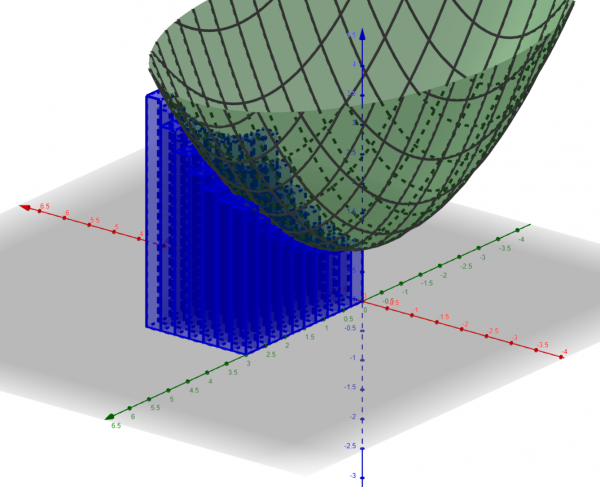A few years ago I got turned on to Geogebra, probably the world’s most awesome and low-barrier-to-entry mathematical software. The best part of Geogebra is that you can do most everything through the web client or the mobile app. (I have the desktop client, but I never use it!)

Here are some doodads I’ve built with Geogebra.

### Visualizing Directional DerivativesThis gadget illustrates one way to think about the directional derivative. There are versions of this same gadget for partial derivatives and the tangent plane, too.

### 3D Riemann Sums over a RectangleA tool for visualizing how the Riemann sums obtained by taking a product partition of the rectangle$[0,2]\times[0,3]$ approximate the volume under the graph. You can specify how many subintervals to partition$[0,2]$ and$[0,3]$ into, and change the function$f(x,y)$.

### 3D Riemann Sums over a Non-Rectangular RegionThe same as the previous applet, but this one is over the region$0\leq y\leq 2$,$0\leq x\leq 3-\frac{3}{4}y^2$.

### Level SetsA tool for visualizing the relationship between the level curves of a function$f(x,y)$, the graph of$f(x,y)$, and the intersection of the graph with horizontal planes.

### The Frenet-Serret FrameYou specify a curve, and this applet animates its Frenet-Serret frame.

### The Matrix of a ReflectionChange the slider to alter the slope of the line; watch as the reflections of the points$(1,0)$ and$(0,1)$ — and the image of Satan from the Codex Gigas — move along with the line. The matrix representing the reflection is obtained from the coordinates of the reflected points.

### Constructing Lines in the Poincaré Disk

This isn’t a Geogebra gadget itself, but it shows how awesome Geogebra is for doing exploratory assignments.